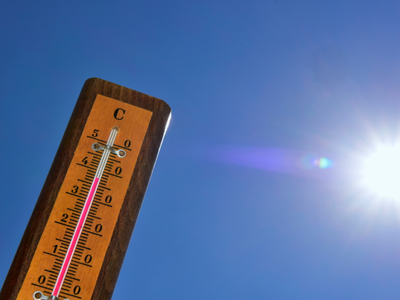One question presents a formula that converts degrees Celsius (centigrade) to degrees Fahrenheit.

# Formulas

This Math quiz is called 'Formulas' and it has been written by teachers to help you if you are studying the subject at high school. Playing educational quizzes is a user-friendly way to learn if you are in the 9th or 10th grade - aged 14 to 16.

It costs only \$12.50 per month to play this quiz and over 3,500 others that help you with your school work. You can subscribe on the page at Join Us

Rearranging complicated formula could be considered as the pinnacle of algebra skills. Are you at the Everest of your algebraic manipulation skills? Find out in this high school Math quiz.

Formulas (or formulae) are very useful in all aspects of life, but particularly to scientists and engineers. Once a formula has been derived, it means that future scientists and engineers don’t have to rediscover it for themselves. If you study Physics you will be familiar with Newton’s second law, F=ma. Imagine if you had to work out this relationship every time you tried to solve a force problem – you would soon not bother!

There are two key skills about using formula. The first is substitution – when you are given the value of a variable, you replace the variable with this number each time it occurs in the formula.

Substitution occurs in many other areas of algebra, so you should already be familiar with it.

The second skill is rearranging a formula. Formulae tend to be stated and remembered in one arrangement. For instance, you should recall that the formula for the volume of a cylinder is V = πr2h. This is fine if you know r and h. But what if you are given the volume, V, and the height, h, and need to find r? You will need to rearrange the formula so that r is the subject. Can you master it?

Question 1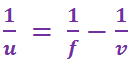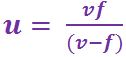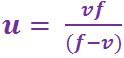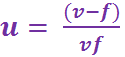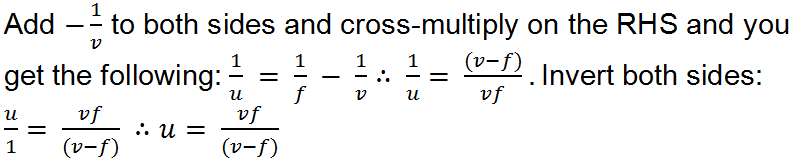Question 2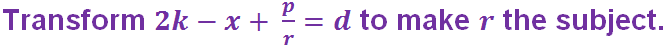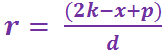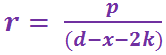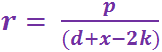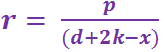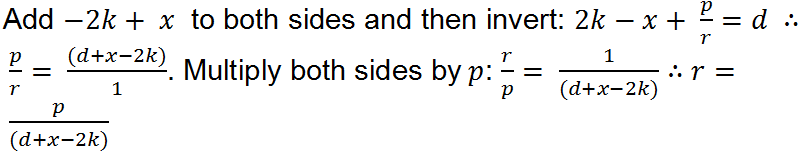Question 3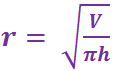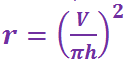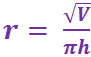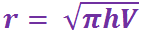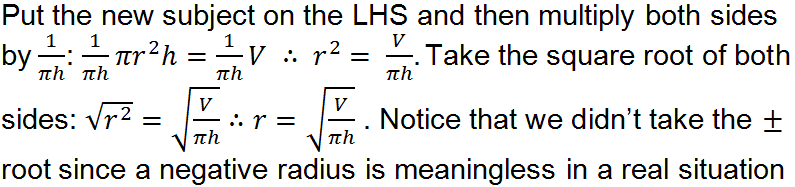Question 4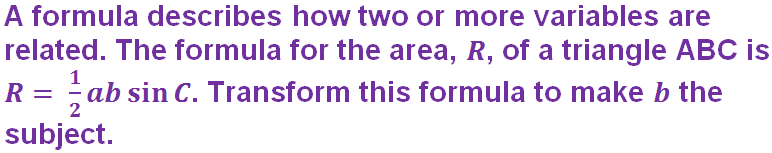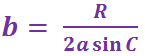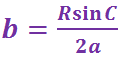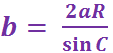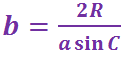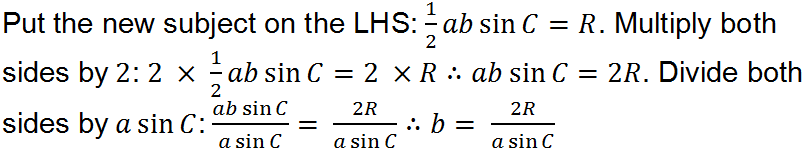Question 5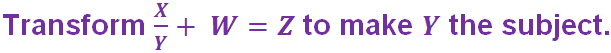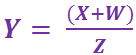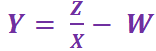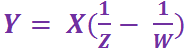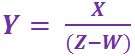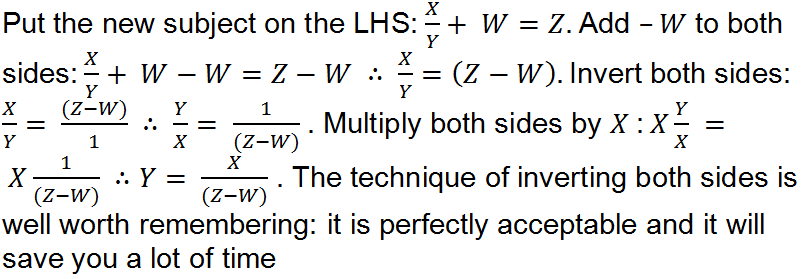Question 6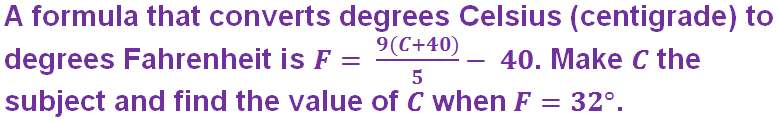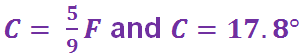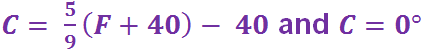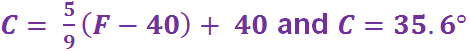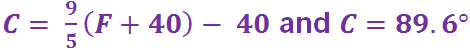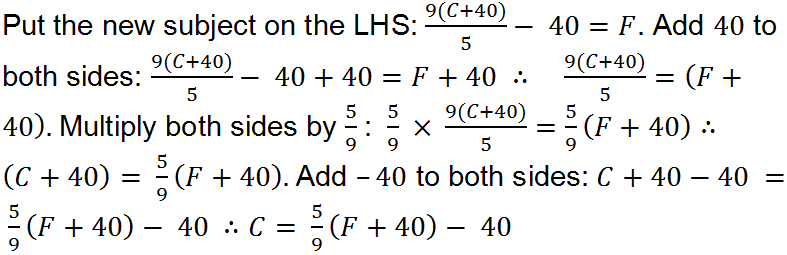Question 7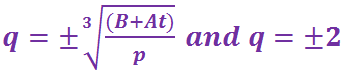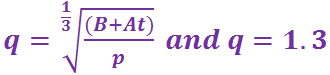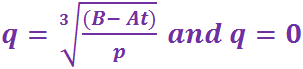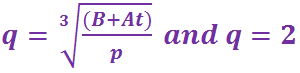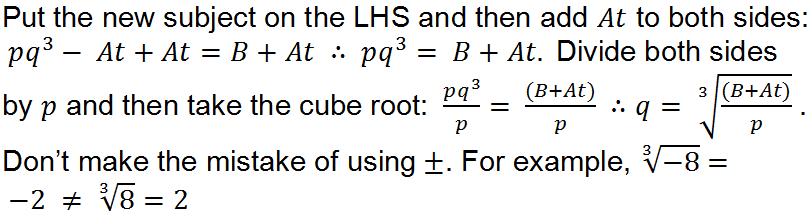Question 8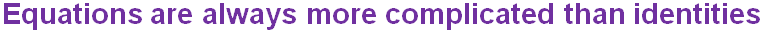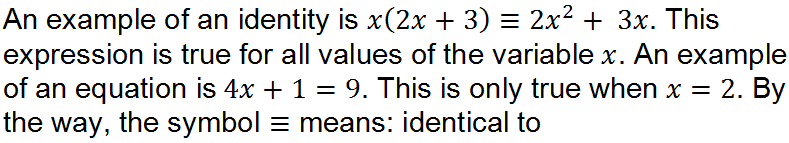Question 9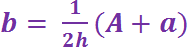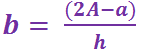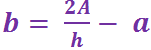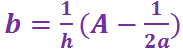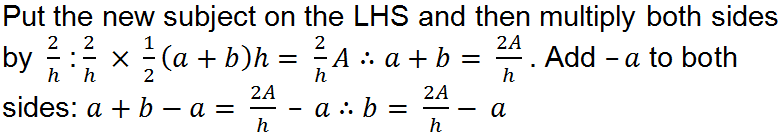Question 10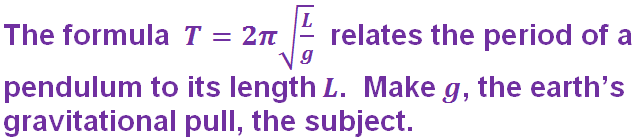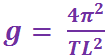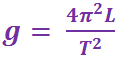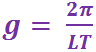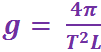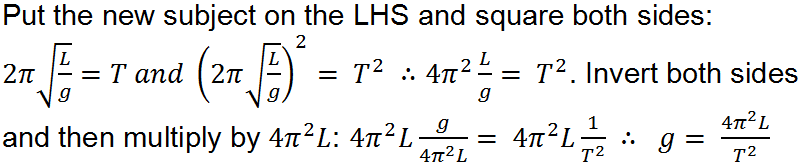Author:  Frank Evans